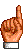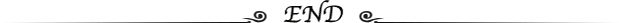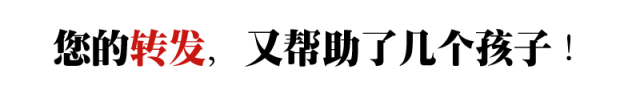# 小学二年级数学上册思维训练题(含答案)

## 欢迎点击上方蓝字免费订阅！

()+()=()+()=()+()

()里最大能填几?

()-9﹤80 80-()﹤20+25

30+()﹤40 26厘米+()厘米﹤1米

()米-80米﹤16米 1米-()厘米﹥12厘米

1. 400-29+362-71+38

2. 399+299+599+199

1.(62)+(38)=(54)+(46)=(27)+(73);

2.36;

3.54;

4.88, 36, 9 , 73 , 95 , 87;

5.5, 5;

6.4;

7.24;

8.5, 4;

9.1, 2;

1.=400+(362+38)-(29+71)

=400+400-100

=700

2.=(400-1)+(300-1)+(600-1)+(200-1)

=400+300+600+200-4

=1496

1.43+2=45(人) 45+4=49(人) 43+45+49=137(人)

2.30-18=12(千克) 18-12=6(千克) 30-6=24(千克)

3.52+46=98(千克) 98-48=50(千克) 50÷2=25(千克)(甲)

52-25=27(千克)(乙) 46-25=21(千克)(丙)

4.15+5+7=27(条) 27÷3=9(条) 9-5=4(条)9-7=2(条)扫二维码免费订阅～###热门文章推荐95.6%的用户看完本文后，还关注了以下精彩公众号